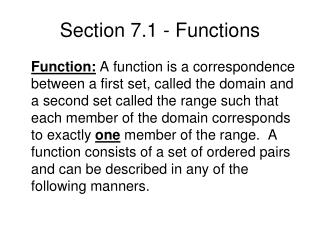DownloadDownload PresentationSection 7.1 - Functions

# Section 7.1 - Functions

Télécharger la présentation## Section 7.1 - Functions

- - - - - - - - - - - - - - - - - - - - - - - - - - - E N D - - - - - - - - - - - - - - - - - - - - - - - - - - -
##### Presentation Transcript

1. Section 7.1 - Functions Function: A function is a correspondence between a first set, called the domain and a second set called the range such that each member of the domain corresponds to exactly one member of the range. A function consists of a set of ordered pairs and can be described in any of the following manners.

2. 1 2 3 4 5 9 13 17 Domain Range 1 2 3 4 5 9 13 17 Domain Range For each problem below list the ordered pairs and indicate if the ordered pairs represent a function.1. Mappings List the ordered pairs.

3. Equations • y = 3x + 2 Identify three ordered pairs Graph your ordered pairs

4. 3. Graphs a. See page 451 problem 26 Complete these ordered pairs:

5. 4. Function Notation: f(x) = 3x - 5 Read: f of x is equal to …., the f(x) symbol replaces the “y” in the equation. This notation can be used to “evaluate” the function and to identify ordered pairs that “fit” the function.

6. a. If f(x) = 3x + 2, Evaluate the linear function and identify the three ordered pairs a. f(2) = b. f(-4) = c. f(0) =

7. b. If g(x) = x2 + 5, Evaluate this polynomial function and identify the three ordered pairs a. g(3) = b. g(-1) = c. g(0)=

8. If evaluate this rational function and identify the three ordered pairs: a. h(7) = b. h(14) = c. h(6)=

9. 5. Graphs of functions: For each graph determine a. f(0), b. f(1), and c. f(-3) See page 451 – problem 28 a. b. c. See Page 451 - problem 30 a. b. c.